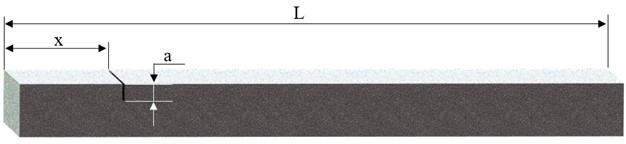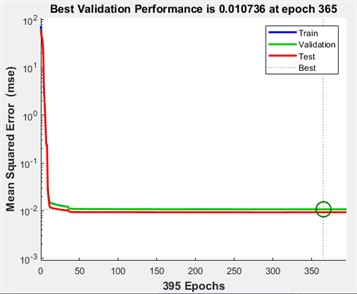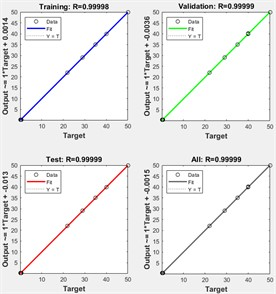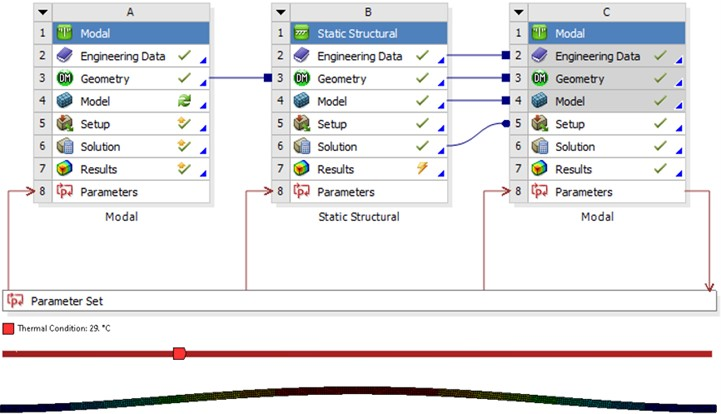Published: 20 October 2023

# Damage detection in variable temperature conditions using artificial intelligence

Alexandra-Teodora Aman1
Cristian Tufisi2
Gilbert-Rainer Gillich3
Tiberiu Manescu4
1, 3, 4Doctoral School of Engineering, Babes-Bolyai University, Cluj-Napoca 400084, Romania
1, 4Department of Automation, Industrial Engineering, Textiles and Transportation, Aurel Vlaicu University, Arad, Romania
2, 3Department of Engineering Science, Babes-Bolyai University, Cluj-Napoca 400084, Romania
Corresponding Author:
Cristian Tufisi
Views 20

#### Abstract

When considering damage detection using the natural frequencies of structures, small frequency drops can indicate either the presence of cracks or a temperature change. This change can lead to additional stress affecting the modal parameters for specific structures, making it much harder to detect, locate, and evaluate damage accurately. The current research aims to describe a method for detecting transverse cracks in beams, considering temperature variations. The considered beam is fixed at both ends, thus inducing axial forces when the temperature is increased. The influence of temperature is considered using adjustment coefficients developed for each vibration mode. This coefficient can be used to accurately calculate the natural frequency for an intact or damaged beam. An analytical method for determining the natural frequencies caused by the changing temperature and the presence of a transverse crack is described and used to generate data for training a feedforward artificial neural network (ANN). The ANN’s capability of determining the position of transverse cracks in double-clamped beams subjected to small temperature changes is proven by creating numerical simulations with known crack positions and thermal conditions for testing the developed method.

## 1. Introduction

Transverse cracks in double-clamped beams can negatively affect their structural integrity, leading to unsafe consequences in various engineering applications. The development of efficient crack detection techniques is of crucial importance . In recent years, researchers have explored different approaches that combine analytical methods and artificial intelligence with the consideration of environmental variations for enhanced crack detection and localization [2-4].

Traditionally, analytical methods have provided valuable insights into the behavior of double-clamped beams subjected to external factors such as cracks . One widely recognized technique employs the squared normalized modal curvature as a reliable indicator of crack presence . This method is based on the fact that cracks modify the modal parameters of the beam, altering its dynamic response. While this analytical approach has proven effective, further improvements are required to enhance detection accuracy and precisely locate the cracks.

To overcome these shortcomings, researchers have turned their attention to artificial intelligence techniques . The concept of vibration-based damage identification comprehends comparing the natural frequencies obtained from the undamaged beam with those from the damaged beam . By employing natural frequencies, even small changes caused by cracks can be detected . Moreover, employing artificial intelligence models allows for more efficient analysis of the measured data, significantly enhancing crack detection accuracy.

In addition to the mentioned techniques, considering temperature variations offers a valuable contribution to the crack detection problem . Temperature changes result in thermal expansion or contraction of the material, imposing additional strains and stresses on the beam . As a result, in practical situations, monitoring structures requires considering both operational and environmental influences . Occasionally, fluctuations in temperature can lead to more significant parameter variations compared to the impact of early-stage damage, making it challenging to differentiate between these two factors . By accounting for temperature variations during the crack detection process, an enhanced understanding of the crack behavior and severity can be achieved with higher accuracy and reliability .

The current study presents an innovative approach to identify transverse breathing cracks in beams under different temperature conditions, utilizing their natural frequencies. The primary issue tackled is the challenge of differentiating minor frequency decreases resulting from cracks from those caused by temperature variations, by using an analytical method that can give an accurate result regarding the impact that transverse cracks have on the structural modal characteristics of beams in varying temperature conditions.

This paper continues the research presented in , aiming to develop an innovative approach to detect and locate transverse cracks in double-clamped steel beams with high accuracy, even when temperature changes occur. Our proposed methodology combines a well-established method  using artificial intelligence models and incorporating temperature adjustment coefficients into the analysis. By employing this comprehensive context, we aim to enhance crack detection sensitivity and localization precision, ensuring the safety and reliability of structural systems.

The developed ANN model accuracy is tested by performing modal simulations for double-clamped steel beams in the simulation software ANSYS, considering known damage scenarios and thermal conditions. The mathematical model

In standard applications, the detection of transverse cracks using modal parameters is approached by assuming unchanged environmental conditions. In real-life cases, the environment is often subjected to changes, which must be considered when applying global damage detection techniques.

Temperature changes affect double-clamped beams most because the constraining conditions lead to supplementary axial loads. In the current study, we employ a method developed in  to calculate the RFS values for damage locations by considering temperature adjustment coefficients. The specimen is a steel beam with its physical properties and dimensions presented in Table 1. The beam is clamped on both ends, and the considered damage is a transverse crack of known depth $a$ and location $x$ as presented in Fig. 1.

Fig. 1Considered damaged beamTable 1The beam’s physical, mechanical, and geometrical parameters

 Mass density $\rho$ [kg/m3] Young modulus $E$ [N/m2] Poisson ratio $\upsilon$ [–] Thermal expansion coefficient $\alpha$ [1/°C] Length [mm] Width [mm] Thickness [mm] 7850 2.1·1011 0.3 1.15·10-5 1000 50 10

If the reference temperature ${T}_{ref}$ is increased with $\mathrm{\Delta }T$, the internal load is given by :

1
$P\left(T\right)=\alpha \cdot E\cdot A\cdot \mathrm{\Delta }T,$

where $A$ is the cross-section of the beam.

The characteristic equation for a beam fixed at both ends is:

2
$\zeta \mathrm{sin}\zeta +2\mathrm{cos}\zeta -2=0.$

The critical forces ${P}_{cr}$ and critical temperature ${T}_{cr}$ have several solutions and can be found as:

3
${P}_{cr-i}=\frac{{{\zeta }_{i}}^{2}E\cdot I}{{L}^{2}},$
4
${T}_{cr-i}={T}_{ref}+\mathrm{\Delta }{T}_{cr}={T}_{ref}+\frac{{P}_{cr-i}}{\alpha \cdot E\cdot A}.$

By applying the transcendental Eq. (5), the values for the first six bending modes are obtained:

5
$1+\mathrm{cos}\lambda \cdot \mathrm{cosh}\lambda =0.$

In the case of compression load, the expression for the case of fixed-fixed ends, for ${\zeta }_{i}\ne {\lambda }_{i}$, the natural frequencies are obtained with Eq. (6):

6
${f}_{i}\left(P\right)={f}_{ref-i}\sqrt{1-\frac{P}{{P}_{cr-i}}}.$

The mathematical relation expressing the frequency considering the temperature change is obtained by substituting Eqs. (1) and (3) in Eq. (6), as:

7
${f}_{i}\left(T\right)={f}_{ref-i}\sqrt{1-\frac{\alpha A\left(T-{T}_{ref}\right){L}^{2}}{I{\zeta }_{i}^{2}}}={f}_{ref-i}\cdot {\kappa }_{i}\left(T\right),$

where ${k}_{i}\left(T\right)$ is the temperature adjustment coefficient. It is employed to find the frequency at any pre-buckling temperature if the frequency at a reference temperature and the temperature change are known.

For a reference temperature ${T}_{ref}$, the first six buckling eigenvalues ${\zeta }_{i}$, critical force ${P}_{cr}$, and critical temperature ${T}_{cr}$ values for the double-clamped beam are shown in Table 2.

Table 2The first six buckling eigenvalues, critical forces, and temperatures

 Buckling mode $i$ Eigenvalue ${\zeta }_{i}$ Critical force ${P}_{cr-i}$ [N] Critical temperature ${T}_{cr-i}$ [°C] 1 6.283185 33885.64 50.607 2 8.986818 69321.50 80.523 3 12.56637 135542.6 136.43 4 15.45050 204899.7 194.98 5 18.84956 304970.8 279.46 6 21.80824 408222.9 366.63

The mathematical equation predicting the natural frequency for a damaged beam subjected to a temperature change is found by applying Eq. (8):

8
${f}_{iD}\left({x}_{D},a,T\right)={f}_{i}\left(T\right)\left\{1-\gamma \left(a\right){\left[{\stackrel{-}{{\varphi }^{\text{'}\text{'}}}}_{i}\left({x}_{D}\right)\right]}^{2}\right\}={f}_{ref-i}\cdot \sqrt{1-\frac{\alpha A\left(T-{{T}_{ref}}_{-i}\right){L}^{2}}{I{\zeta }_{i}^{2}}}\cdot \left\{1-\gamma \left(a\right){\left[{\stackrel{-}{{\varphi }^{\text{'}\text{'}}}}_{i}\left({x}_{D}\right)\right]}^{2}\right\}.$

The severity $\gamma \left(a\right)$ for a given crack depth $a$ can be found by applying the method presented in , and the squared normalized modal curvature for the double-clamped beam can be found using Eq. (9):

9
${{\varphi }^{\text{'}\text{'}}}_{i}\left(x\right)=\left(\mathrm{cos}{\lambda }_{i}\frac{x}{L}+\mathrm{cosh}{\lambda }_{i}\frac{x}{L}\right)-\frac{\left(\mathrm{sin}{\lambda }_{i}+\mathrm{sinh}{\lambda }_{i}\right)}{\left(\mathrm{cos}{\lambda }_{i}-\mathrm{cosh}{\lambda }_{i}\right)}\left(\mathrm{sin}{\lambda }_{i}\frac{x}{L}+\mathrm{sinh}{\lambda }_{i}\frac{x}{L}\right).$

## 2. Training the ANN with the mathematical model

The ANN is trained to evaluate the first six weak-axis bending natural frequency data obtained from a double-clamped steel beam subjected to various temperature conditions (ranging from 22 to 50 °C) while it is also affected by a transverse breathing crack. This section details the method for generating the training data for the ANN using the proposed algorithm. The training data is obtained by calculating the natural frequencies for a double-clamped beam, starting from a reference temperature ${T}_{ref}=$22 °C, for which Eq. 10 is used:

10
${f}_{i}=\frac{{\lambda }_{i}^{2}}{2\pi }\sqrt{\frac{EI}{\rho A{L}_{}^{4}}}.$

Furthermore, we consider several thermal scenarios to depict the thermal adjustment coefficients. These imply four temperature increase values, i.e., $\mathrm{\Delta }T=$7, 13, 18, and 28 °C. The obtained coefficients are presented in Table 3.

 Temperature increase $\mathrm{\Delta }T$ [°C] ${k}_{1}$(T) ${k}_{2}$(T) ${k}_{3}$(T) ${k}_{4}$(T) ${k}_{5}$(T) ${k}_{6}$(T) 29 0.86909 0.93829 0.96893 0.97956 0.98631 0.98979 35 0.73863 0.88197 0.94148 0.96169 0.97443 0.98096 40 0.60893 0.83213 0.91799 0.94654 0.96441 0.97354 50 0.14573 0.72219 0.86908 0.91549 0.94406 0.14573

After obtaining the ${k}_{i}\left(T\right)$ coefficients, we calculate the frequency for each temperature for the intact beam by using Eq. (7). Next, we calculate the frequency for a damaged beam again for each selected temperature using Eq. (8). The damage is a transverse crack, that is removed with a step of 2 mm along the hall length of the beam. The crack depth is 2 mm, meaning a cross-section reduction of 20 %. The severity value $\gamma \left(a\right)$ has been obtained by applying the procedure described in . Due to the symmetrical constraining condition, the training data is calculated for only half of the beam. Thus, two possible damage locations can be considered as the predicted position, starting from the left or right sides of the beam. It results in 1250 training scenarios.

Fig. 2ANN training performancea) Performance diagramb) Regression diagrams

The ANN is a feedforward neural network with two hidden layers, each containing 50 neurons, and is developed using MATLAB software. The network is trained to predict the location of the crack and the temperature of the beam, using the trainBR function. After the training is completed, the first network evaluation is done by analyzing the performance and regression diagrams, shown in Fig. 2.

The diagrams in Fig. 2 illustrate that the trained ANN is well-equipped to handle the complexity of transverse crack localization in a varying temperature environment.

## 3. Testing the ANN using ANSYS simulations and thermal transient analysis

The validation of the developed ANN for transverse crack localization in a double-clamped steel beam, having its dimensions presented in Table 1, subjected to varying temperature conditions, is performed using simulated damage scenarios defined within ANSYS software.

These scenarios included the introduction of a 2 mm depth transverse crack into the beam structure and encompassed a range of temperatures from 22 to 44 °C. To accurately simulate real-world conditions, the models employ the thermal transient method to integrate the effects of thermal stresses induced by axial forces resulting from temperature fluctuations starting from ${T}_{ref}=$22 °C. Modal analysis was employed to extract the first six weak-axis eigenfrequencies of the damaged beam under different temperature conditions. The analysis setup is presented in Fig. 3.

The modal analysis results, consisting of the natural frequencies, served as the basis for feature extraction in the ANN testing process. This information was fed into the trained ANN model, allowing the network to predict the presence and location of the transverse crack for each simulation scenario.

Fig. 3Simulation setupThe results obtained for each damage scenario are presented in Table 4. From the results, one can observe that the maximum difference between the real and the predicted crack position is 42 mm, for small structures this error is negligible, but for larger structures, the method should be further improved by developing more complex ANN structures and by normalizing the data.

## 4. Conclusions

While the accuracy of the developed Artificial Neural Network (ANN) for transverse crack detection in a double-clamped steel beam under varying temperature conditions might not have reached optimal levels, the error being over 3 % for damage cases 6, 8, 11, 14 in the current study, the research provides a solid foundation for future advancements. Incorporating thermal stresses induced by temperature fluctuations into the ANN testing process represented a significant step toward real-world applicability. Despite the challenges, the study showcases the potential of integrating modal analysis results into ANN-based damage detection frameworks, showing that in some cases, the damage location has been predicted with an error of 1 mm.

Table 4Obtained results

 Scenario No. Damage location $\mathrm{x}$ [m] Temperature [°C] Predicted location $\mathrm{x}$ [m] Error[%] Predicted temperature [°C] 1 0.02 29 0.024 0.40 31.70 2 0.031 35 0.021 1.00 34.09 3 0.046 40 0.050 0.40 41.79 4 0.058 44 0.059 0.10 48.45 5 0.063 29 0.040 2.30 31.76 6 0.089 35 0.059 3.00 34.05 7 0.169 40 0.201 3.20 41.48 8 0.294 44 0.253 4.10 48.33 9 0.562 29 0.558 0.40 29.06 10 0.625 35 0.649 2.40 33.92 11 0.789 40 0.747 4.20 41.38 12 0.802 44 0.799 0.30 48.28 13 0.226 37 0.249 2.30 36.96 14 0.192 26 0.227 3.50 23.21 15 0.058 25 0.04 1.80 22.22

One promising way for future research lies in exploring the utilization of relative frequency shifts. A more sensitive and accurate detection method can be developed by examining the relative changes in eigenfrequencies due to transverse cracks. This approach could enhance the ANN's ability to discern subtle differences in the structural behavior caused by cracks, especially under varying thermal conditions.

02 October 2023
Accepted
16 October 2023
Published
20 October 2023
SUBJECTS
Mathematical models in engineering
Keywords
crack localization
double-clamped beam
natural frequency
artificial intelligence
temperature variations
Acknowledgements

The authors have not disclosed any funding.

Data Availability

The datasets generated during and/or analyzed during the current study are available from the corresponding author on reasonable request.

Conflict of interest

The authors declare that they have no conflict of interest.